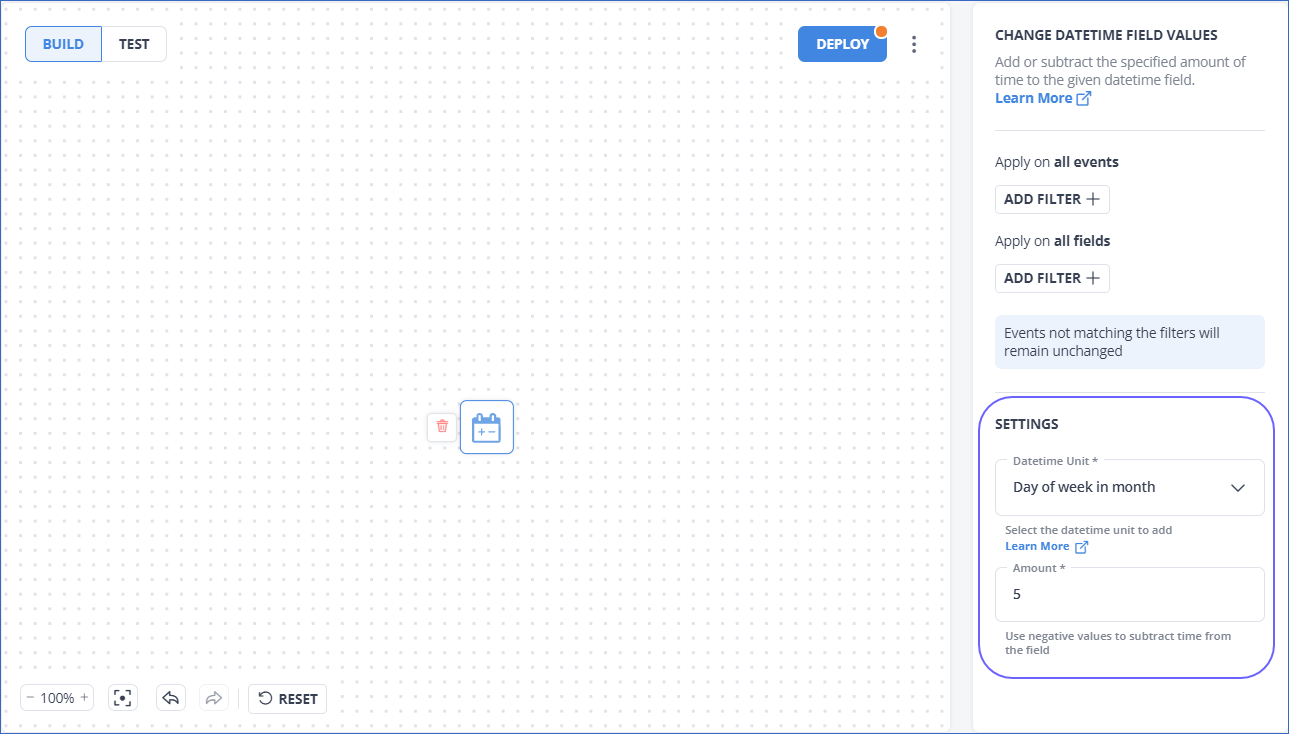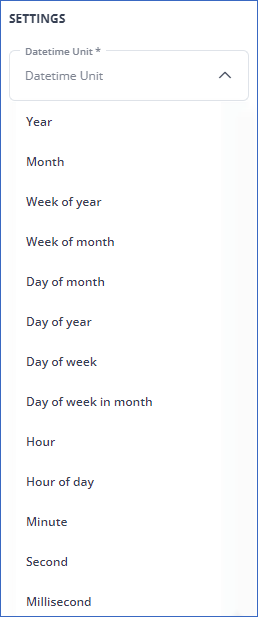# Datetime Field Values

This section describes the DateTime units that you can use to provide the settings in the Change DateTime Field Values Transformation.Note: A positive date-time value is added whereas a negative value is subtracted from the existing field value.

The following image illustrates the Datetime units that Hevo currently supports:Refer to the following table to know more about each Datetime unit:

DateTime Unit Description
Year Represents the year value of a date-time field.
Its value starts from 1.
Month Represents the month value of a date-time field.
Its value starts from 0, indicating the month of January.
Week of year Represents the week number within the current year.
Its value starts from 1, indicating the first week of the year.
Week of month Represents the week number within the current month.
Its value starts from 1, indicating the first week of the month.
Day of month Represents the day number within the current month.
Its value starts from 1, indicating the first day of the month.
Day of year Represents the day number within the current year.
Its value starts from 1. For example, the Day of year for 5th February equals 36.
Day of week Represents the day of a week.
Its value starts from 1, indicating the first day of the week, Sunday.
Day of week in month Represents the ordinal number of the week of the month in which the day falls.
Its value starts from 1. Day 1 of the current month is treated as the start of the week, and all days within a given week return the same value. For example, the day of week in month for 1st to 7th of March is 1, for 8th to 14th of March it is 2, and so on.
Hour of day Represents the hour of the day in a 24-hour clock.
Its value ranges between 0 and 23.
Minute Represents the minute within the current hour.
Its value ranges between 0 and 59.
Second Represents the second within the current minute.
Its value ranges between 0 and 59.
Millisecond Represents milliseconds within the second.
Its value ranges between 0 and 999.

## Revision History

- Removed the `Hour` DateTime Unit as it is merged with the `Hour of day` DateTime Unit.